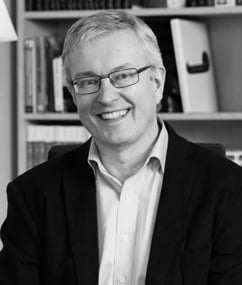# Background information and assumptions for Ecophon Acoustic Calculator

The parameters are defined in ISO 3382 part 1 and 2. The Sabine formula is used for calculation of reverberation times under the assumption of a diffuse sound field. The reverberation times given by the Sabine formula are used to estimate speech clarity and sound strength under diffuse conditions.

In rooms where the main part of the absorption is located in the ceiling, e.g. as a suspended sound-absorbing ceiling, the assumption of a diffuse sound field is not valid and the assumptions for the Sabine formula are not fulfilled. In this calculator a model especially adopted for rooms with sound-absorbing ceilings is used for this type of room.

The basic ideas behind the model are presented in . For rooms where the dominating part of the sound absorption is due to the sound-absorbing ceiling, the non-diffuse model gives estimations of T20, C50 and G that better corresponds to measured values, than estimations based on Sabine’s formula.

Generally, in rooms with acoustic ceiling treatment, the Sabine calculations gives shorter reverberation times compared to what is actually measured.

## Assumptions concerning the calculations

#### When non-diffuse calculations and when Sabine calculations?

Both Sabine and non-diffuse calculations are performed for all cases with a full absorbent ceiling. A full sound-absorbing ceiling means a coverage range of at least 85% of the soffit area. Soffit refers to the surface above the suspended ceiling.

When the ceiling coverage range is less than 85% only Sabine calculation is performed.

Calculations with free-hanging units or baffles are only performed with the Sabine formula since the non-diffuse model is not applicable for these cases.The calculations only takes the total area of the wall absorbers added to the room into considerations. The acoustic effect of furniture is quantified in the measure equivalent scattering absorption area Asc.

#### What is calculated?

Reverberation time T20 (s), Speech Clarity C50 (dB) and Sound Strength G (dB) are calculated both for diffuse and non-diffuse conditions. The parameters are defined in the standards ISO 3382 part 1 and 2. The calculated values are estimations of what can be expected from measurements when the measured parameters are averaged over the floor area.

Concerning wall absorbers, the calculations takes only into account the total area of the wall absorbers added to the room. The distribution on the different walls is not accounted for.

#### What about the suspended ceiling?

It is assumed that the suspended ceiling always is parallel with the floor. For the case with the sloped soffit an average mounting height is calculated.

#### What about furniture and interior equipment?

The acoustic effect of furniture is quantified in the measure equivalent scattering absorption area Asc. Values are given for furniture configurations corresponding to sparse, normal and dense furnishing.

The limited choices of background absorption for walls, soffit, floor, windows and doors are motivated by the relatively small influence of this absorption compared to the influence of the sound-absorbing ceiling, wall absorbers and furniture. The selected material covers what is typically encountered in practise.

#### What about the solution for extra low-frequency absorption?

The Ecophon Extra Bass is an encapsulated light-weight glass wool product to be placed above a suspended ceiling thus improving the low-frequency absorption. Our recommendation is that a 50% coverage is enough for satisfactory results. However, in the calculations a 100% coverage is assumed.

The power attenuation coefficient in air (m) is from EN 12354-6:2003 and for 20° C and 50–70% humidity.

 Frequency (Hz) 125 250 500 1000 2000 4000 m (1/m) 0.0001 0.0003 0.0006 0.001 0.0017 0.0041

####What about the model?

The model is developed by Erling Nilsson, PhD, Acoustics Specialist at Ecophon and Adjunct Professor at the Department of Engineering Acoustics at Lund University.

More detailed information about the model is to be found below in .

The development has been conducted in close cooperation with the Technical University of Denmark (DTU) , .

1) Nilsson E. Input data for acoustical design calculations for ordinary public rooms, ICSV24, July 2017, London

2) G. Marbjerg, J. Brunskog, C.-H. Jeong, and E. Nilsson, Development and validation of a combined phased acoustical radiosity and image source model for predicting sound fields in rooms, J. Acoust. Soc. Am. 138, 1457–1468 (2015).

3) Bakoulas Konstantinos, Optimization of an energy-based room acoustics model that considers scattering and non-uniform absorption, Master thesis, Department of Electrical Engineering, Technical University of Denmark, July, 2017